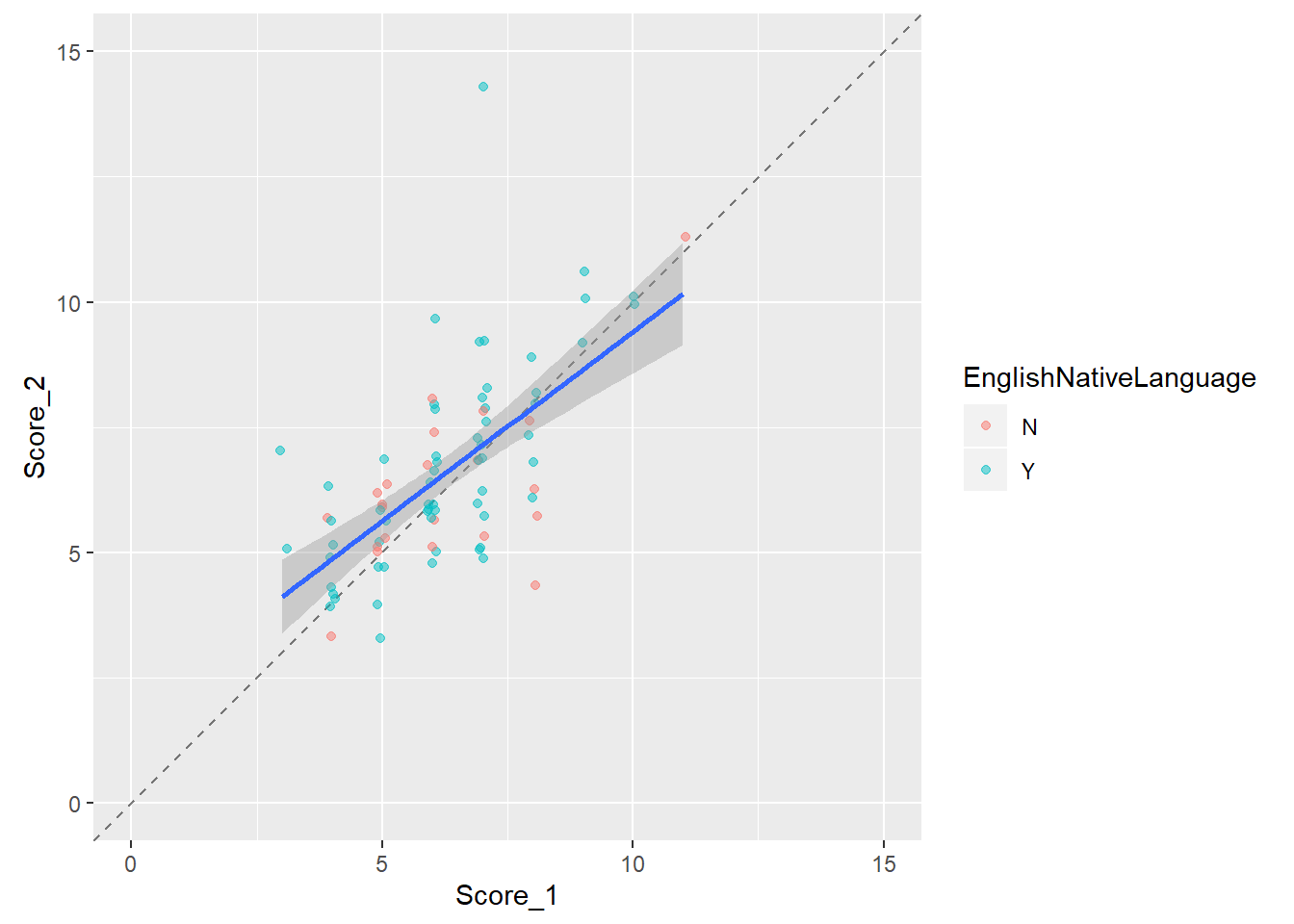Include your answers in this document in the sections below the rubric.

# Rubric

1. (1 p) Participate in two data collection and entering activities. Describe any potential issues about the data collection process.

2. (2 p) Interpret correlation for Males, Females, and Everyone combined.

3. (1 p) How would the correlation change if both hand span and height were measured in inches?

4. (2 p) Why is there a difference in the strength of the correlation for everyone compared to either gender separately?

5. (2 p) Describe the relationships between the scores and the guessed score.

6. (2 p) Identify and explain the most surprising feature of these data.

# Height vs Hand Span

Procedure:

1. Record your height in inches. For example 5’0" is 60 inches.
2. Use a ruler to measure your hand span in centimeters: the distance from the tip of your thumb to pinky finger with your hand splayed as wide as possible.
4. Analysis.

## Data and Plots

library(tidyverse)
## -- Attaching packages ---------------------------------------- tidyverse 1.2.1 --
## v ggplot2 3.2.1     v purrr   0.3.2
## v tibble  2.1.3     v dplyr   0.8.3
## v tidyr   1.0.0     v stringr 1.4.0
## v readr   1.3.1     v forcats 0.4.0
## -- Conflicts ------------------------------------------- tidyverse_conflicts() --
## x dplyr::lag()    masks stats::lag()
# install.packages("gsheet")

# Height vs Hand Span
library(gsheet)
dat_hand <-
gsheet2tbl(dat_hand_url) %>%
as.data.frame() %>%
na.omit() %>%
mutate(
Gender_M_F = factor(Gender_M_F, levels = c("F", "M"))
)

str(dat_hand)
## 'data.frame':    82 obs. of  5 variables:
##  $Table : num 1 1 1 2 2 2 2 2 2 3 ... ##$ Person     : num  1 2 3 1 2 3 4 5 6 1 ...
##  $Gender_M_F : Factor w/ 2 levels "F","M": 2 1 2 1 1 1 2 2 2 1 ... ##$ Height_in  : num  66 65 74.5 64.5 60 63 74 69 67 63 ...
##  $HandSpan_cm: num 21 19 23.5 19.5 17.5 17.5 23.5 21 23 19.5 ... # Plot the data using ggplot and ggpairs library(ggplot2) library(GGally) ## Registered S3 method overwritten by 'GGally': ## method from ## +.gg ggplot2 ## ## Attaching package: 'GGally' ## The following object is masked from 'package:dplyr': ## ## nasa p1 <- ggpairs(dat_hand %>% select(Gender_M_F, Height_in, HandSpan_cm) , mapping = ggplot2::aes(colour = Gender_M_F) , lower = list(continuous = "smooth") , diag = list(continuous = "density") #, upper = list(params = list(corSize = 6)) ) ## Warning in check_and_set_ggpairs_defaults("diag", diag, continuous = ## "densityDiag", : Changing diag$continuous from 'density' to 'densityDiag'
print(p1)
## stat_bin() using bins = 30. Pick better value with binwidth.
## stat_bin() using bins = 30. Pick better value with binwidth.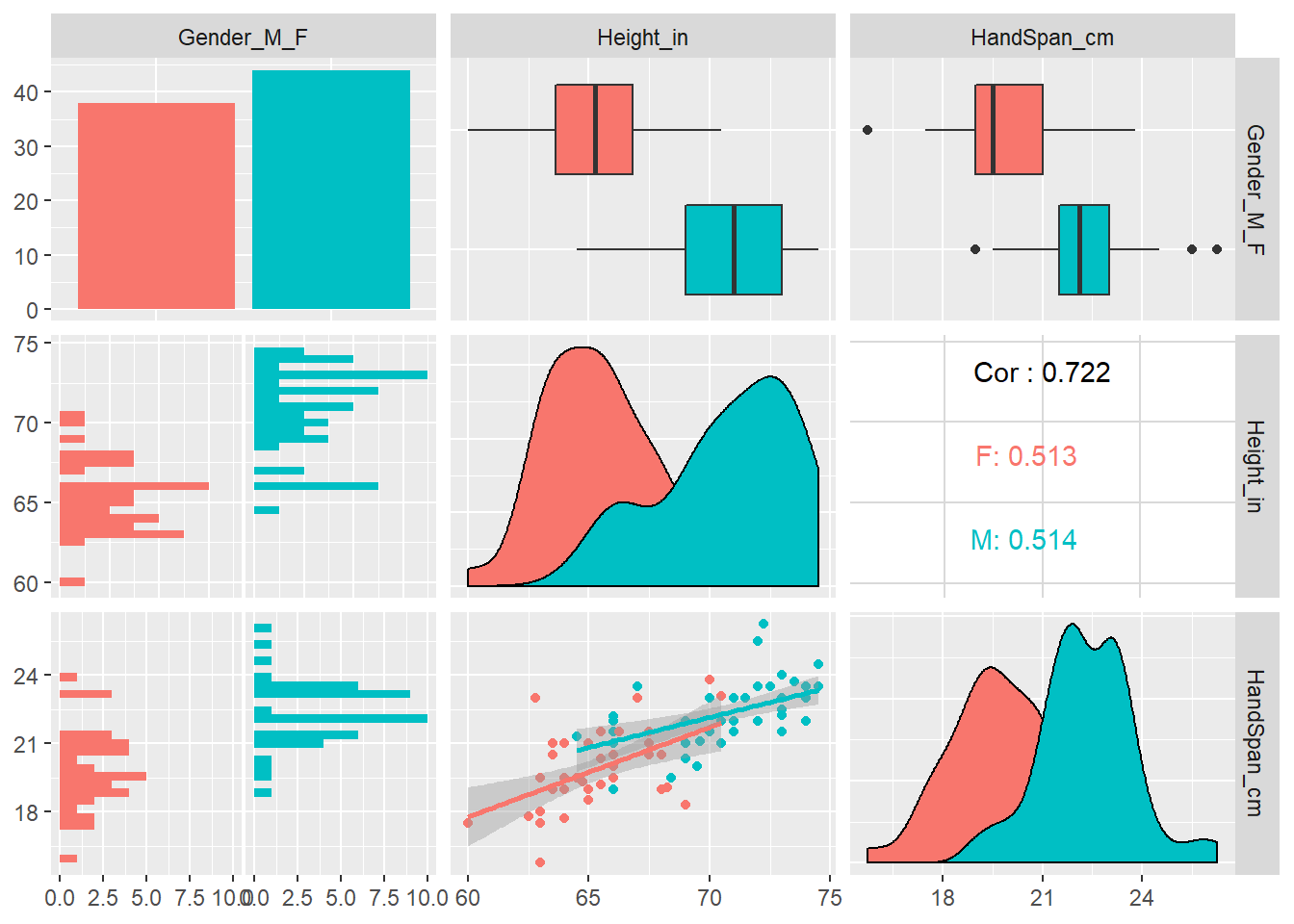• Describe any potential issues about the data collection process.

• Interpret correlation for Males, Females, and Everyone combined.

• How would the correlation change if both hand span and height were measured in inches?

• Why is there a difference in the strength of the correlation for everyone compared to either gender separately?

# Word memory scores

15 seconds to memorize 15 words: http://www.randomlists.com/random-words?qty=15

Procedure:

1. Round 1
1. Put up a list of words for 15 seconds and view.
2. Have 60 seconds to write/type as many words as you can remember.
3. Score yourself (anonymous, so honesty is best – we’re all going to be bad at this).
2. Given your first performance, make a guess at how many words you’ll remember in round 2.
3. Round 2 (repeat of round 1)
5. Analysis.

## Data and Plots

# Memory Scores
library(gsheet)
dat_memory <-
gsheet2tbl(dat_memory_url) %>%
as.data.frame() %>%
na.omit() %>%
mutate(
Gender_M_F            = factor(Gender_M_F, levels = c("F", "M"))
, EnglishNativeLanguage = factor(EnglishNativeLanguage)
)
str(dat_memory)
## 'data.frame':    83 obs. of  8 variables:
##  $Table : num 1 1 1 1 1 2 2 2 2 2 ... ##$ Person               : num  1 2 3 8 9 1 2 3 4 5 ...
##  $Gender_M_F : Factor w/ 2 levels "F","M": 2 1 2 2 1 1 1 1 2 2 ... ##$ UGrad_Grad           : Factor w/ 2 levels "G","U": 2 2 2 1 1 2 2 2 2 2 ...
##  $EnglishNativeLanguage: Factor w/ 2 levels "N","Y": 1 1 1 2 1 1 2 2 2 1 ... ##$ Score_1              : num  5 6 6 7 7 8 7 6 8 8 ...
##  $Guessed_2 : num 5 6 6 7 9 7 8 6 10 8 ... ##$ Score_2              : num  6 7 6 7 7 8 5 6 9 6 ...
# Plot the data using ggplot and ggpairs
library(ggplot2)
library(GGally)
, lower = list(continuous = "smooth")
, diag  = list(continuous = "density")
#, upper = list(params = list(corSize = 6))
)
## Warning in check_and_set_ggpairs_defaults("diag", diag, continuous =
## "densityDiag", : Changing diag\$continuous from 'density' to 'densityDiag'
print(p2)
## stat_bin() using bins = 30. Pick better value with binwidth.
## stat_bin() using bins = 30. Pick better value with binwidth.
## stat_bin() using bins = 30. Pick better value with binwidth.
## stat_bin() using bins = 30. Pick better value with binwidth.
## stat_bin() using bins = 30. Pick better value with binwidth.
## stat_bin() using bins = 30. Pick better value with binwidth.
## stat_bin() using bins = 30. Pick better value with binwidth.
## stat_bin() using bins = 30. Pick better value with binwidth.
## stat_bin() using bins = 30. Pick better value with binwidth.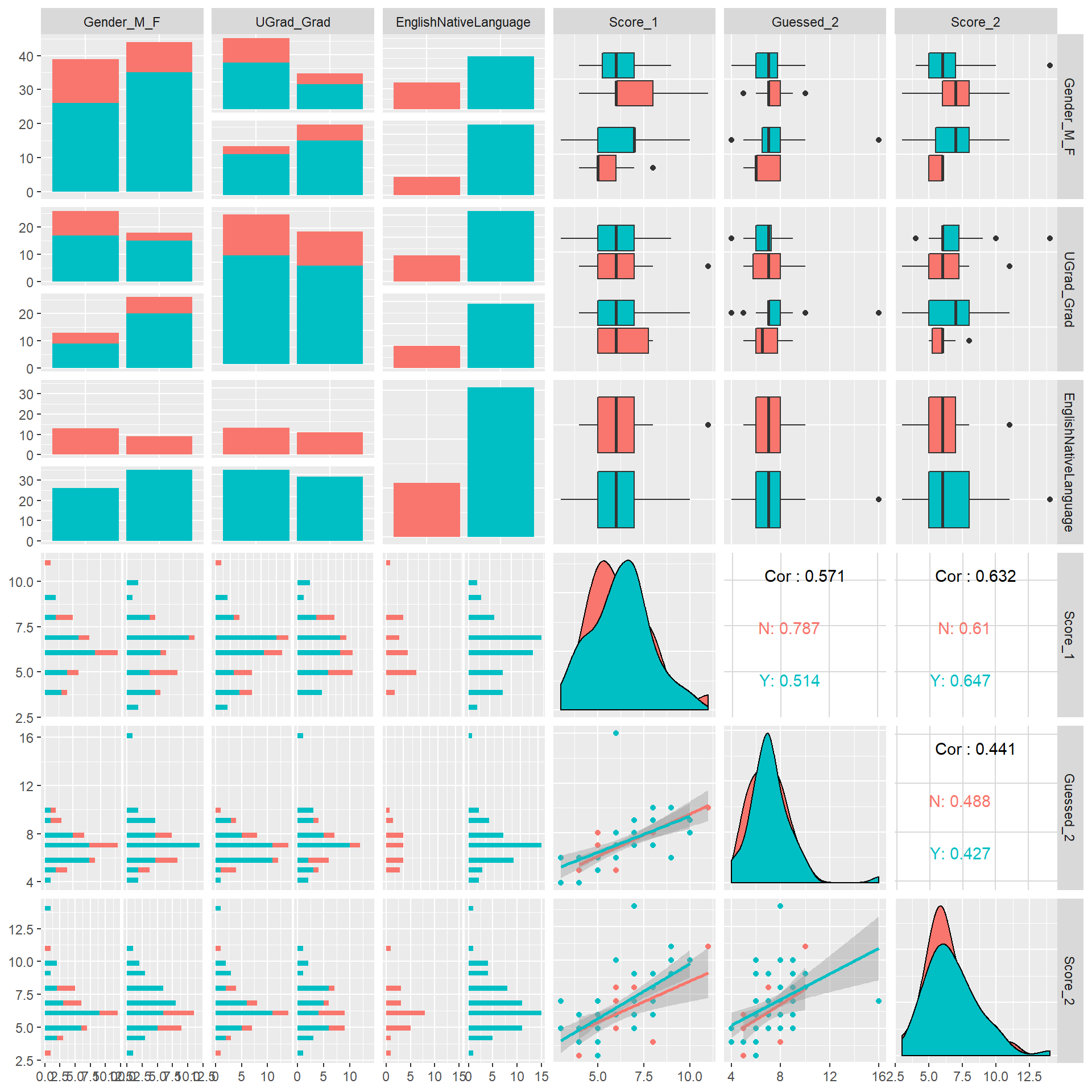library(ggplot2)
p <- ggplot(dat_memory, aes(x = Score_1, y = Guessed_2))
p <- p + geom_abline(intercept = 0, slope = 1, linetype = "dashed", alpha = 0.5)
p <- p + geom_jitter(aes(colour = EnglishNativeLanguage), position = position_jitter(width = 0.1), alpha = 1/2)
p <- p + geom_smooth(method = lm)
p <- p + scale_y_continuous(limits=c(0, 15))
p <- p + scale_x_continuous(limits=c(0, 15))
p <- p + coord_fixed(ratio = 1)
print(p)
## Warning: Removed 1 rows containing non-finite values (stat_smooth).
## Warning: Removed 1 rows containing missing values (geom_point).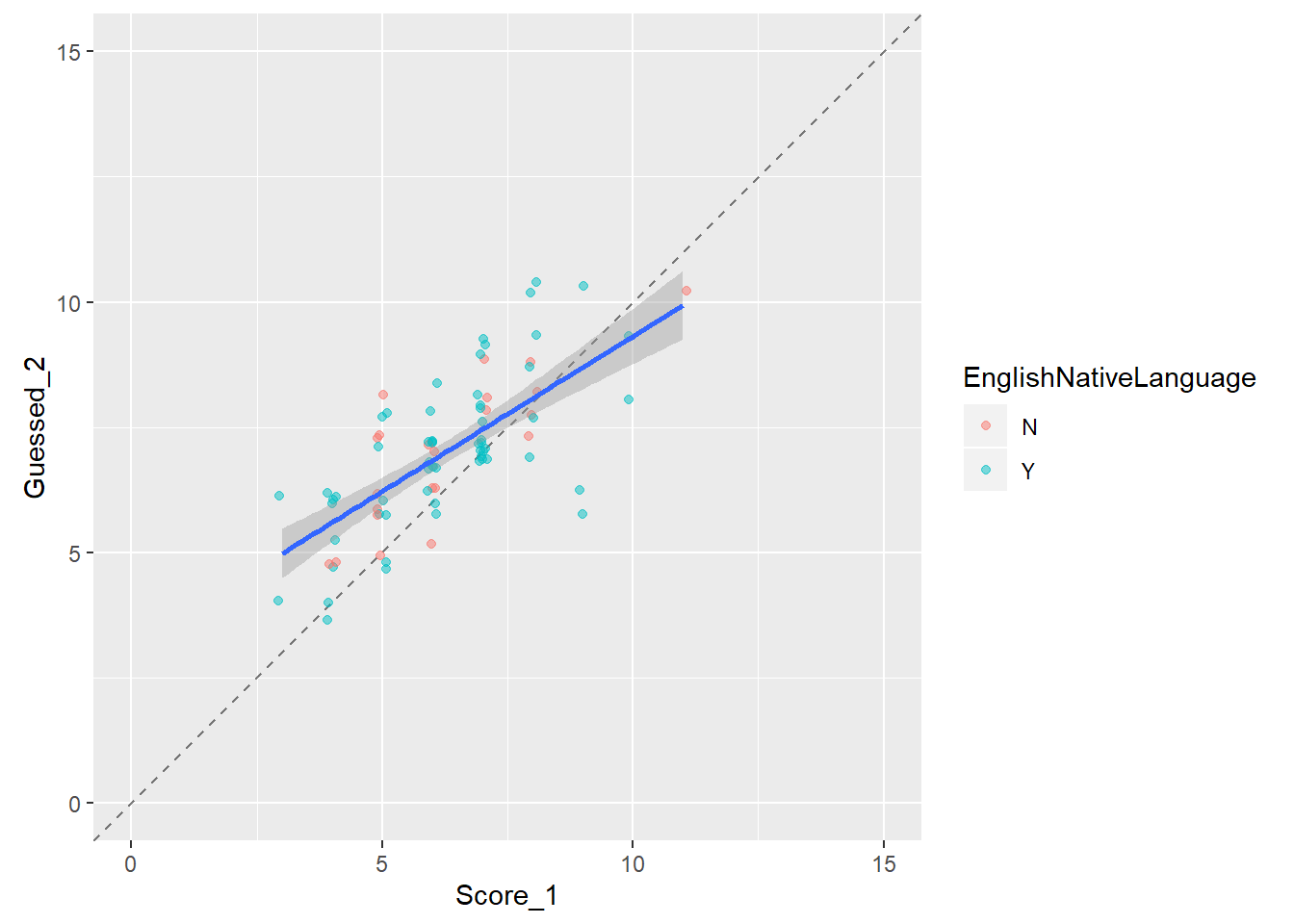library(ggplot2)
p <- ggplot(dat_memory, aes(x = Guessed_2, y = Score_2))
p <- p + geom_abline(intercept = 0, slope = 1, linetype = "dashed", alpha = 0.5)
p <- p + geom_jitter(aes(colour = EnglishNativeLanguage), position = position_jitter(width = 0.1), alpha = 1/2)
p <- p + geom_smooth(method = lm)
p <- p + scale_y_continuous(limits=c(0, 15))
p <- p + scale_x_continuous(limits=c(0, 15))
p <- p + coord_fixed(ratio = 1)
print(p)
## Warning: Removed 1 rows containing non-finite values (stat_smooth).

## Warning: Removed 1 rows containing missing values (geom_point).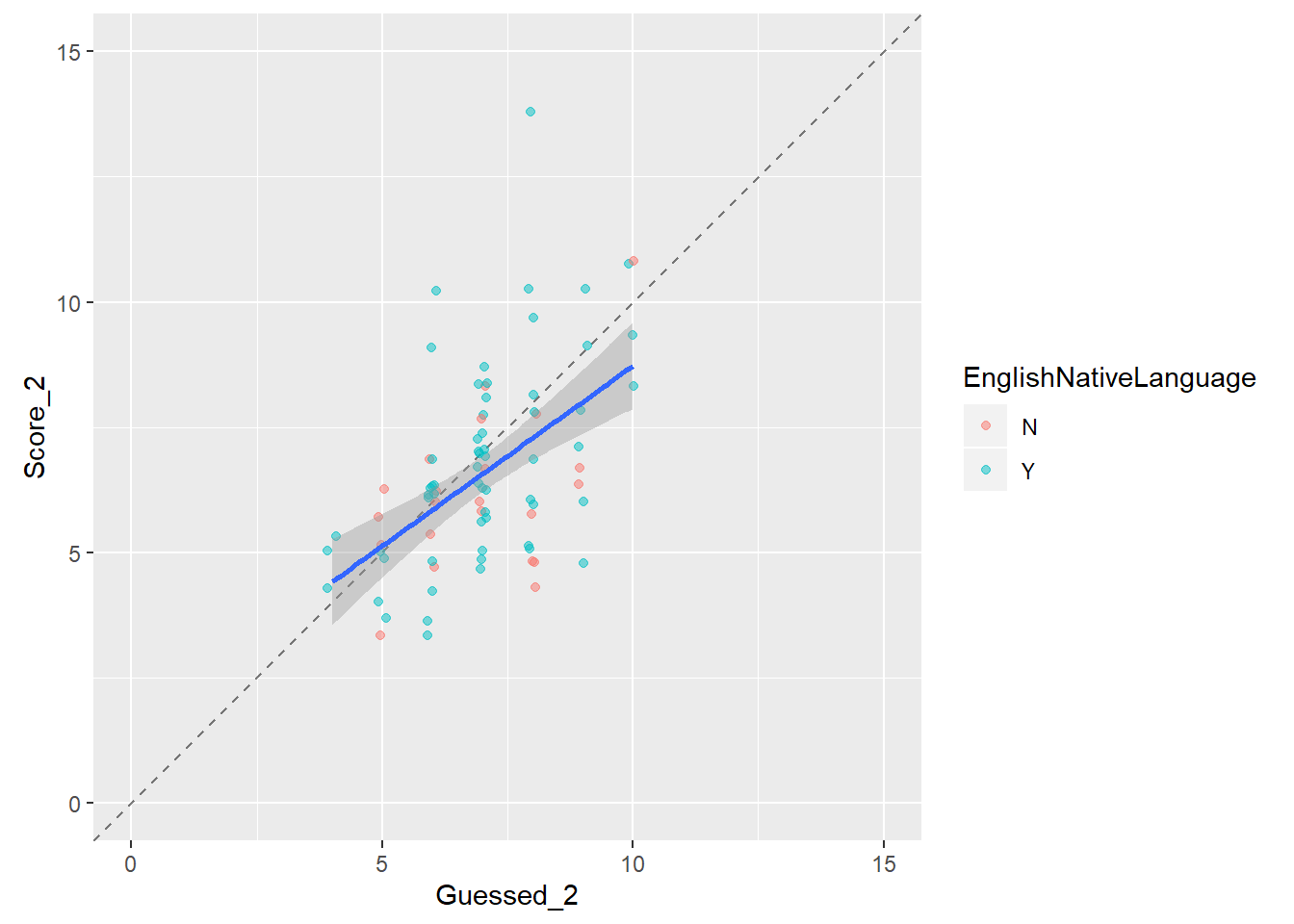library(ggplot2)
p <- ggplot(dat_memory, aes(x = Score_1, y = Score_2))
p <- p + geom_abline(intercept = 0, slope = 1, linetype = "dashed", alpha = 0.5)
p <- p + geom_jitter(aes(colour = EnglishNativeLanguage), position = position_jitter(width = 0.1), alpha = 1/2)
p <- p + geom_smooth(method = lm)
p <- p + scale_y_continuous(limits=c(0, 15))
p <- p + scale_x_continuous(limits=c(0, 15))
p <- p + coord_fixed(ratio = 1)
print(p)